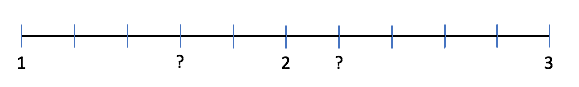# Practice – Place a fraction on the number line

Questions :

Question 1

Study the number line. Write the fraction where you see a “?”.Question 2

Study the number line. Write the fraction where you see a “?”.Question 2

Study the number line. Write the fraction where you see a “?”.Question 3

Draw a number line and place the following fractions on the number line.Question 4

Draw a number line and place the following fractions on the number line.Question 5

Draw a number line and place the following fractions on the number line.Explanations :Video Explanation :

Question 2Video Explanation :

Question 3

Video Explanation :

Question 4

Video Explanation :

Question 5

Video Explanation :

Scroll to Top